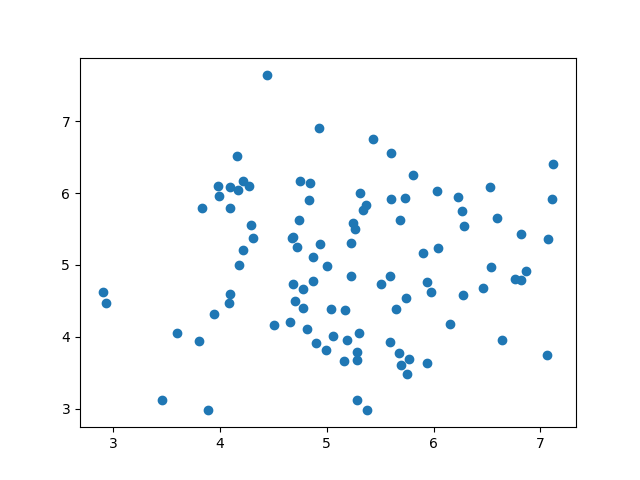## Python Tutorial

Python HOME Python Intro Python Get Started Python Syntax Python Comments Python Variables Python Data Types Python Numbers Python Casting Python Strings Python Booleans Python Operators Python Lists Python Tuples Python Sets Python Dictionaries Python If...Else Python While Loops Python For Loops Python Functions Python Lambda Python Arrays Python Classes/Objects Python Inheritance Python Iterators Python Scope Python Modules Python Dates Python Math Python JSON Python RegEx Python PIP Python Try...Except Python User Input Python String Formatting

## File Handling

Python File Handling Python Read Files Python Write/Create Files Python Delete Files

## Python Modules

NumPy Tutorial Pandas Tutorial SciPy Tutorial Django Tutorial

## Python Matplotlib

Matplotlib Intro Matplotlib Get Started Matplotlib Pyplot Matplotlib Plotting Matplotlib Markers Matplotlib Line Matplotlib Labels Matplotlib Grid Matplotlib Subplot Matplotlib Scatter Matplotlib Bars Matplotlib Histograms Matplotlib Pie Charts

## Machine Learning

Getting Started Mean Median Mode Standard Deviation Percentile Data Distribution Normal Data Distribution Scatter Plot Linear Regression Polynomial Regression Multiple Regression Scale Train/Test Decision Tree Confusion Matrix Hierarchical Clustering Logistic Regression Grid Search Categorical Data K-means Bootstrap Aggregation Cross Validation AUC - ROC Curve K-nearest neighbors

## Python MySQL

MySQL Get Started MySQL Create Database MySQL Create Table MySQL Insert MySQL Select MySQL Where MySQL Order By MySQL Delete MySQL Drop Table MySQL Update MySQL Limit MySQL Join

## Python MongoDB

MongoDB Get Started MongoDB Create Database MongoDB Create Collection MongoDB Insert MongoDB Find MongoDB Query MongoDB Sort MongoDB Delete MongoDB Drop Collection MongoDB Update MongoDB Limit

## Python Reference

Python Overview Python Built-in Functions Python String Methods Python List Methods Python Dictionary Methods Python Tuple Methods Python Set Methods Python File Methods Python Keywords Python Exceptions Python Glossary

## Module Reference

Random Module Requests Module Statistics Module Math Module cMath Module

## Python How To

Remove List Duplicates Reverse a String Add Two Numbers

## Python Examples

Python Examples Python Compiler Python Exercises Python Quiz Python Certificate

# Machine Learning - Scatter Plot

## Scatter Plot

A scatter plot is a diagram where each value in the data set is represented by a dot.The Matplotlib module has a method for drawing scatter plots, it needs two arrays of the same length, one for the values of the x-axis, and one for the values of the y-axis:

`x = [5,7,8,7,2,17,2,9,4,11,12,9,6]`

`y = [99,86,87,88,111,86,103,87,94,78,77,85,86]`

The `x` array represents the age of each car.

The `y` array represents the speed of each car.

### Example

Use the `scatter()` method to draw a scatter plot diagram:

import matplotlib.pyplot as plt

x = [5,7,8,7,2,17,2,9,4,11,12,9,6]
y = [99,86,87,88,111,86,103,87,94,78,77,85,86]

plt.scatter(x, y)
plt.show()

### Result:Run example »

### Scatter Plot Explained

The x-axis represents ages, and the y-axis represents speeds.

What we can read from the diagram is that the two fastest cars were both 2 years old, and the slowest car was 12 years old.

Note: It seems that the newer the car, the faster it drives, but that could be a coincidence, after all we only registered 13 cars.

## Random Data Distributions

In Machine Learning the data sets can contain thousands-, or even millions, of values.

You might not have real world data when you are testing an algorithm, you might have to use randomly generated values.

As we have learned in the previous chapter, the NumPy module can help us with that!

Let us create two arrays that are both filled with 1000 random numbers from a normal data distribution.

The first array will have the mean set to 5.0 with a standard deviation of 1.0.

The second array will have the mean set to 10.0 with a standard deviation of 2.0:

### Example

A scatter plot with 1000 dots:

import numpy
import matplotlib.pyplot as plt

x = numpy.random.normal(5.0, 1.0, 1000)
y = numpy.random.normal(10.0, 2.0, 1000)

plt.scatter(x, y)
plt.show()

### Result:Run example »

### Scatter Plot Explained

We can see that the dots are concentrated around the value 5 on the x-axis, and 10 on the y-axis.

We can also see that the spread is wider on the y-axis than on the x-axis.